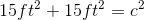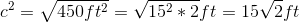# ISEE Upper Level Math : How to find the length of the diagonal of a square

## Example Questions

### Example Question #1 : How To Find The Length Of The Diagonal Of A Square

What is the diagonal of a square with a side of 4?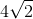Explanation:

Squares have all congruent sides. To find the diagonal, first recognize that you're dealing with an isoceles triangle when you draw the diagonal in the square. That means that two of the sides are congruent in the triangle. Thus, it's a special 45-45-90 triangle. In such triangles, the sides are x and the hypotenuse is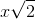. Since we know x is 4, we can plug in 4 to the expression. Thus, the answer is.

### Example Question #2 : How To Find The Length Of The Diagonal Of A Square

You recently bought some special filter paper for a laboratory apparatus. The paper comes in square sheets, but you want to cut it into two equal triangle-shaped pieces. If the square sheets have a side length of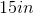, what will the length of the hypotenuse of the triangles be?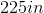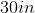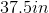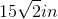Explanation:

You recently bought some special filter paper for a laboratory apparatus. The paper comes in square sheets, but you want to cut it into two equal triangle-shaped pieces. If the square sheets have a side length of, what will the length of the hypotenuse of the triangles be?

This problem is trying to distract you by thinking of triangles. What we are really asked to find here is the length of the diagonal of a square with sides of 15 inches.

Splitting a square along its diagonal yields two 45/45/90 triangles. If you know the ratios for 45/45/90 triangles, you can find the answer very quickly.

Think: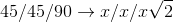Meaning that if the two short sides are x units long, the hypotenuse will be x times the square root of two units long.

In our current case, our short sides are 15 inches long, so our hypotenuse will beYou could also solve this with Pythagorean Theorem.a and b are both 15 in, so we can solve.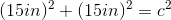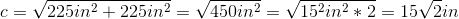### Example Question #3 : How To Find The Length Of The Diagonal Of A Square

While out walking, you find a strange, square-shaped piece of metal. If the side length of the piece is 26 inches, what is the length of the diagonal?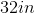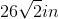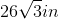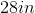Explanation:

While out walking, you find a strange, square-shaped piece of metal. If the side length of the piece is 26 inches, what is the length of the diagonal?

To find the diagonal of a square, we can recognize one of two things.

1) The diagonal of a square creates a right triangle, and we can use Pythagorean theorem to find our diagonal.

2) The diagonal of a square creates two 45/45/90 triangles, with side length ratios of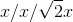Using 2), we can find that the diagonal of the square must be### Example Question #4 : How To Find The Length Of The Diagonal Of A Square

Your new friend has a very small, square-shaped dorm room. She tells you that it is only 225 square feet. Assuming this is true, what is the diagonal distance from one corner of her room to the other?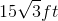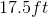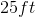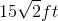Explanation:

Your new friend has a very small, square-shaped dorm room. She tells you that it is only 225 square feet. Assuming this is true, what is the diagonal distance from one corner of her room to the other?

So, we need to find the diagonal of a square. First, we need to find the side length.

Let's begin with our formula for the area of a square: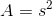where s is our side length and A is our area.

With this formula, we can solve for our side length by plugging in our area and square rooting both sides.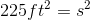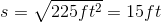Now, to find the diagonal, we can think of an isosceles right triangle, where the two equal sides are 15 ft. This is also a 45/45/90 triangle, which means the side lengths follow the ratio of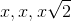This means our answer is.

We could also find this by using Pythagorean Theorem.